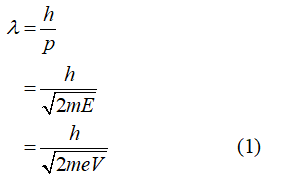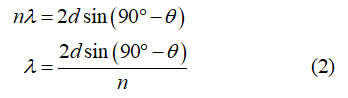# Davisson and Germer scattered electrons from a crystal of nickel. The scattered electrons formed a strong diffraction pattern. What important conclusion was drawn from this experiment?

Question
1 views

Davisson and Germer scattered electrons from a crystal of nickel. The scattered electrons formed a strong diffraction pattern. What important conclusion was drawn from this experiment?

check_circle

Step 1

The Davisson and Germer experiment states that the phase difference of the waves, that are reflected from two different atomic layers of a crystal of the nickel, is fixed. These waves interfere either constructively or destructively after reflection. This results in producing a diffraction pattern.

In this experiment, the waves are used in place of electrons that form a diffraction pattern. Thus, it verifies the dual nature of matter. It can be related to the de Broglie equation and Bragg’s law.

Write the de Broglie equation.Step 2

From the above expression, the wavelength of the wave can be calculated if the voltage is known.

Write the Bragg’s law equation....

### Want to see the full answer?

See Solution

#### Want to see this answer and more?

Solutions are written by subject experts who are available 24/7. Questions are typically answered within 1 hour.*

See Solution
*Response times may vary by subject and question.
Tagged in